# Contents

## Bicoherence

``````from spectrum import bicoherence

bicoherence(y, nfft=None, wind=None, nsamp=None, overlap=None)``````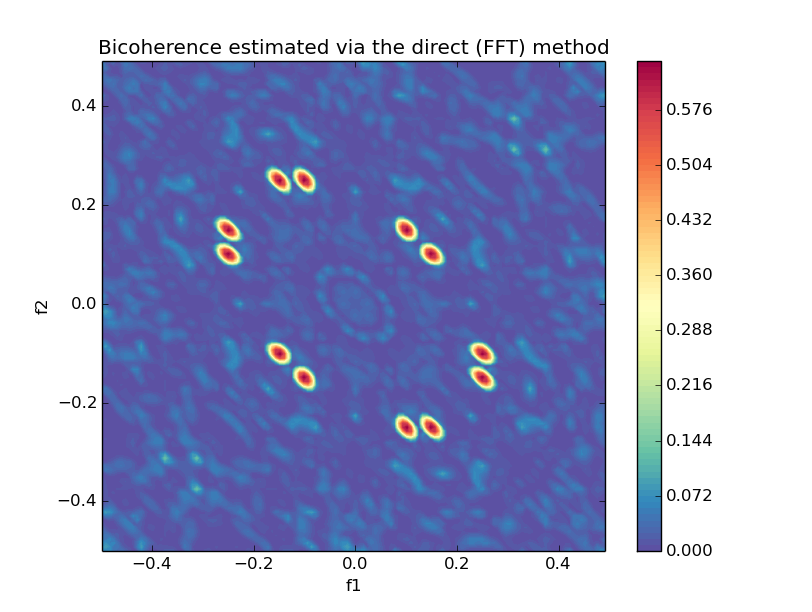## Cross Bicoherence

``````from spectrum import bicoherencex

bicoherencex(w, x, y, nfft=None, wind=None, nsamp=None, overlap=None)``````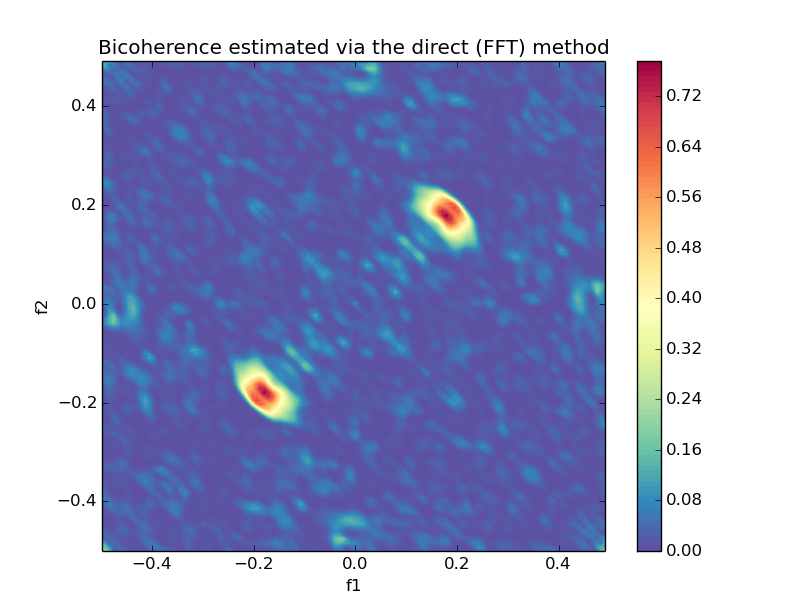## Bispectrum Direct (using fft)

``````from spectrum import bispectrumd

bispectrumd(y, nfft=None, wind=None, nsamp=None, overlap=None)``````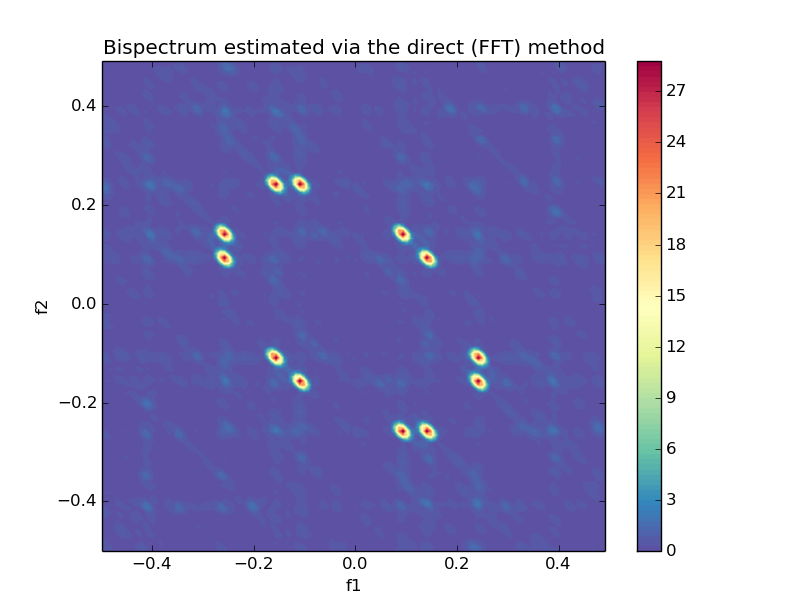## Bispectrum Indirect

``````from spectrum import bispectrumi

bispectrumi(y, nlag=None, nsamp=None, overlap=None, flag='biased', nfft=None, wind=None)``````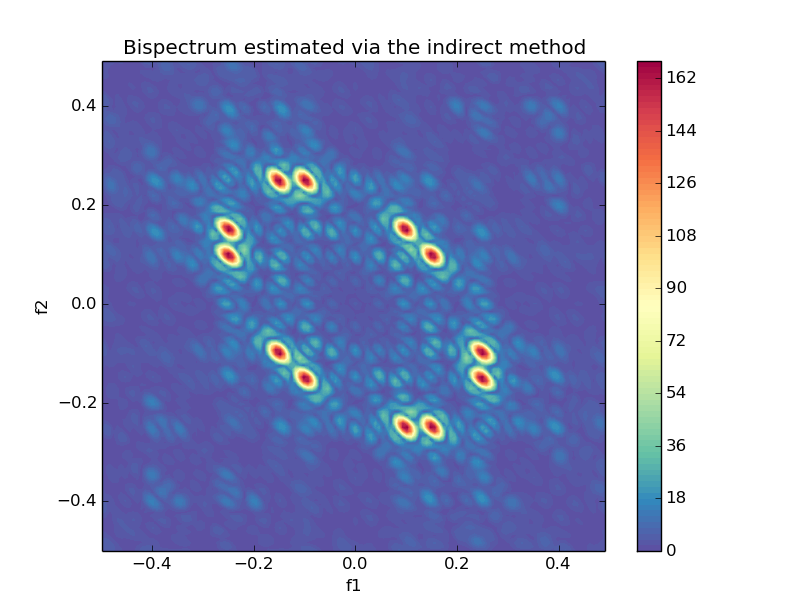## Cross Bispectrum (Direct)

``````from spectrum import bispectrumdx

bispectrumdx(x, y, z, nfft=None, wind=None, nsamp=None, overlap=None)``````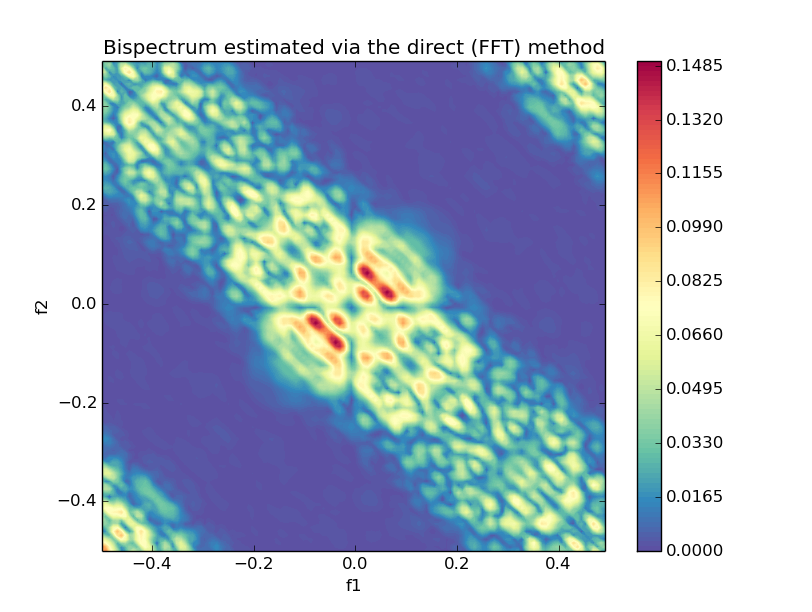## 2nd, 3rd and 4th order cumulants

``````from spectrum import cumest

order = 2 # 2nd order
cumest(y, norder=order, maxlag=0 ,nsamp=None, overlap=0, flag='biased' ,k1=0, k2=0)``````

## 2nd, 3rd and 4th order cross-cumulants

``````from spectrum import cum2x, cum3x, cum4x

cum2x(x, y, maxlag=0, nsamp=0, overlap=0, flag='biased')
cum3x(x, y, z, maxlag=0, nsamp=0, overlap=0, flag='biased', k1=0)
cum4x(w, x, y, z, maxlag=0, nsamp=0, overlap=0, flag='biased', k1=0, k2=0)``````

More coming soon

Taken from the Higher Order Spectral Analysis toolkit for MATLAB